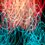# Ring-ing in the rational numbers

Recall that a rational number is a number that can be written as $\frac{a}{b}$, where $a$ and $b$ are integers.

We will explore some properties of rational numbers.

1) The sum of 2 rational numbers is always rational.

Proof: Let the 2 numbers be $\frac{a}{b}$ and $\frac{c}{d}$, where $a, b, c, d$ are integers. Then, their sum is $\frac{ ad+bc}{bd}$, and $ad+bc$ and $bd$ are both integers. Hence, this number is rational.

2) The product of 2 irrational numbers does not need to be irrational.

Proof: In the previous post, we showed the $\sqrt{2}$ is irrational. The product of $\sqrt{2}$ and $\sqrt{2}$ is 2, which is rational.

3) The sum of a rational number and an irrational number is always irrational.

Proof: Let the rational number be $x = \frac{a}{b}$ and the irrational number be $y$. We will prove this statement by contradiction. Suppose that their sum is rational, of the form $\frac{ c}{d}$, then we know that $\frac{a}{b} + y = \frac{c}{d}$, or that $y = \frac{ c}{d} - \frac{a}{b} = \frac{ cb-ad} { bd}$, which is rational. This contradicts the condition that $y$ is irrational. Hence the sum is always irrational.

A) What do we know about the product of 2 rational numbers? Is it always rational?

B) What do we know about the sum of 2 irrational numbers? Is it always irrational?

C) What do we know about the product of a rational number and an irrational number? Is it always irrational? [Hint: Be very careful!]

Can someone give me feedback? Is this too hard for Cosines group, or just right? Do you want to see more basic material?Note by Chung Kevin
6 years, 11 months ago

This discussion board is a place to discuss our Daily Challenges and the math and science related to those challenges. Explanations are more than just a solution — they should explain the steps and thinking strategies that you used to obtain the solution. Comments should further the discussion of math and science.

When posting on Brilliant:

• Use the emojis to react to an explanation, whether you're congratulating a job well done , or just really confused .
• Ask specific questions about the challenge or the steps in somebody's explanation. Well-posed questions can add a lot to the discussion, but posting "I don't understand!" doesn't help anyone.
• Try to contribute something new to the discussion, whether it is an extension, generalization or other idea related to the challenge.

MarkdownAppears as
*italics* or _italics_ italics
**bold** or __bold__ bold
- bulleted- list
• bulleted
• list
1. numbered2. list
1. numbered
2. list
Note: you must add a full line of space before and after lists for them to show up correctly
paragraph 1paragraph 2

paragraph 1

paragraph 2

[example link](https://brilliant.org)example link
> This is a quote
This is a quote
    # I indented these lines
# 4 spaces, and now they show
# up as a code block.

print "hello world"
# I indented these lines
# 4 spaces, and now they show
# up as a code block.

print "hello world"
MathAppears as
Remember to wrap math in $$ ... $$ or $ ... $ to ensure proper formatting.
2 \times 3 $2 \times 3$
2^{34} $2^{34}$
a_{i-1} $a_{i-1}$
\frac{2}{3} $\frac{2}{3}$
\sqrt{2} $\sqrt{2}$
\sum_{i=1}^3 $\sum_{i=1}^3$
\sin \theta $\sin \theta$
\boxed{123} $\boxed{123}$

Sort by:

A) Yes, since a/b x c/d = ac/bd which is rational B) No, consider root2 '+ (1-root2) = 1, which is rational C) No, since 0 is a rational number, which when multiplied by anything gives 0, which is rational

- 6 years, 11 months ago

Wait, is A) true or false?

- 6 years, 11 months ago

Sorry, I meant it is. ac/bd is rational.

- 6 years, 11 months ago

I found this entertaining and basic enough. It introduces the reader into think about how to formulate basic proofs.

- 6 years, 11 months ago

For CosinesGroup, I think that the material should be slightly more basic, in terms of what a 13-14 year old would typically have access to. To me, this would be on the higher end of Cosinesgroup, or even in Torquegroup.

I liked your "Matchstick puzzles" post, and I think posts similar to that will be appropriate.

Staff - 6 years, 11 months ago